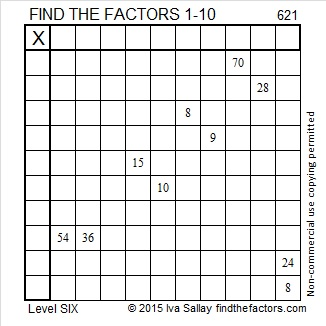# 621 and Level 6

Since 23 x 27 = 621, and both of those factors are 2 away from their average, 25, we know that 621 is only 2^2 numbers away from 25^2. Mathematically we write (25-2)(25+2 ) = (25^2) – (2^2) or 23 x 27 = 625 – 4 = 621.

621 is sandwiched between 595 and 630, the 34th and the 35th triangular numbers. It can be written as the sum of consecutive numbers 7 different ways. The numbers in bold are in the exact middle of each sum. What is the relationship between the numbers in bold and the amount of numbers in the sums?

1. 310 + 311 = 621 ( 2 consecutive numbers because 621 is divisible by 1, but not by 2)
2. 206 + 207 + 208 = 621 (3 consecutive numbers because it is divisible by 3)
3. 101 + 102 + 103 + 104 + 105 + 106 + 107 = 621 (6 consecutive numbers because it is divisible by 3, but not by 2)
4. 65 + 66 + 67 + 68 + 69 + 70 + 71 + 72 + 73 = 621 (9 consecutive numbers because it is divisible by 9)
5. 26 + 27 + 28 + 29 + 30 + 31 + 32 + 33 + 34 + 35 + 36 + 37 + 38 + 39 + 40 + 41 + 42 + 43 = 621 (18 consecutive numbers because it is divisible by 9, but not by 2)
6. 16 + 17 + 18 + 19 + 20 + 21 + 22 + 23 + 24 + 25 + 26 + 27 + 28 + 29 + 30 + 31 + 32 + 33 + 34 + 35 + 36 + 37 + 38 = 621 (23 consecutive numbers because it is divisible by 23)
7. 10 + 11 + 12 + 13 + 14 + 15 + 16 + 17 + 18 + 19 + 20 + 21 + 22 + 23 + 24 + 25 + 26 + 27 + 28 + 29 + 30 + 31 + 32 + 33 + 34 + 35 + 36 = 621 (27 consecutive numbers because it is divisible by 27)Print the puzzles or type the solution on this excel file: 10 Factors 2015-09-14

—————————————————————————————————

• 621 is a composite number.
• Prime factorization: 621 = 3 x 3 x 3 x 23, which can be written 621 = (3^3) x 23
• The exponents in the prime factorization are 3 and 1. Adding one to each and multiplying we get (3 + 1)(1 + 1) = 4 x 2 = 8. Therefore 621 has exactly 8 factors.
• Factors of 621: 1, 3, 9, 23, 27, 69, 207, 621
• Factor pairs: 621 = 1 x 621, 3 x 207, 9 x 69, or 23 x 27
• Taking the factor pair with the largest square number factor, we get √621 = (√9)(√69) = 3√69 ≈ 24.91987.—————————————————————————————————